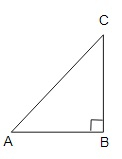# Foot of a $10 \mathrm{~m}$ long ladder leaning against a vertical wall is $6 \mathrm{~m}$ away from the base of the wall. Find the height of the point on the wall where the top of the ladder reaches.

Given:

Foot of a $10 \mathrm{~m}$ long ladder leaning against a vertical wall is $6 \mathrm{~m}$ away from the base of the wall.

To do:

We have to find the height of the point on the wall where the top of the ladder reaches.

Solution:Let $\mathrm{AB}$ be a vertical wall and $\mathrm{AC}$ be a ladder.

$AC=10\ m$

The top of the ladder reaches $\mathrm{A}$ and the distance of the ladder from the base of the wall $B C$ is $6 \mathrm{~m}$.

In right angled triangle $A B C$,

$A C^{2}=A B^{2}+B C^{2}$

$(10)^{2}=A B^{2}+(6)^{2}$

$100=A B^{2}+36$

$A B^{2}=100-36$

$AB^2=64$

$A B=\sqrt{64}$

$AB=8 \mathrm{~m}$

Hence, the height of the point on the wall where the top of the ladder reaches is $8 \mathrm{~m}$.